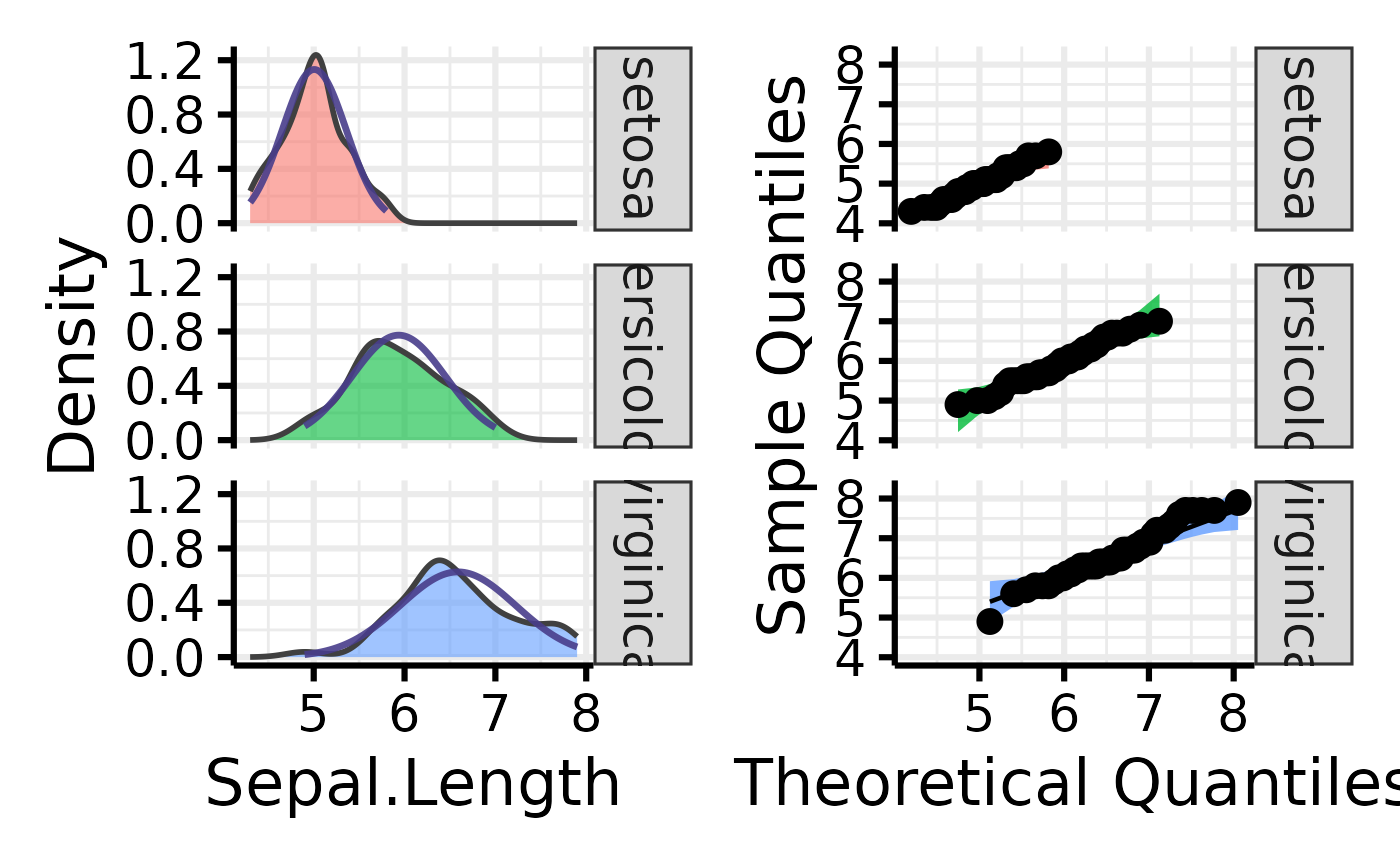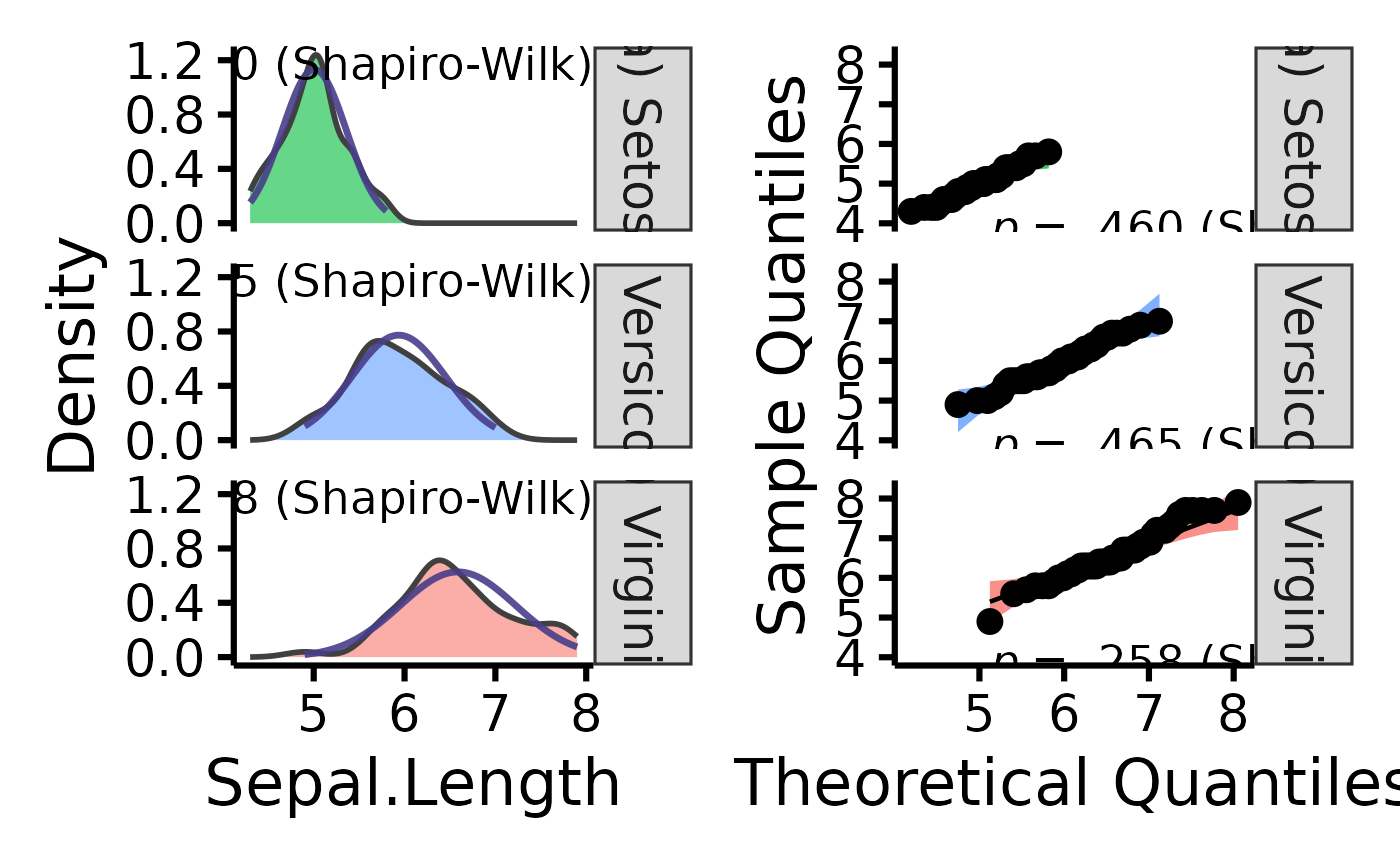Easily make nice per-group density and QQ plots through a wrapper around the ggplot2 and qqplotr packages.

## Usage

nice_normality(
data,
variable,
group = NULL,
colours,
groups.labels,
grid = TRUE,
shapiro = FALSE,
title = NULL,
histogram = FALSE,
breaks.auto = FALSE,
...
)

## Arguments

data

The data frame.

variable

The dependent variable to be plotted.

group

The group by which to plot the variable.

colours

Desired colours for the plot, if desired.

groups.labels

How to label the groups.

grid

Logical, whether to keep the default background grid or not. APA style suggests not using a grid in the background, though in this case some may find it useful to more easily estimate the slopes of the different groups.

shapiro

Logical, whether to include the p-value from the Shapiro-Wilk test on the plot.

title

An optional title, if desired.

histogram

Logical, whether to add an histogram on top of the density plot.

breaks.auto

If histogram = TRUE, then option to set bins/breaks automatically, mimicking the default behaviour of base R hist() (the Sturges method). Defaults to FALSE.

...

Further arguments from nice_qq() and nice_density() to be passed to nice_normality()

## Value

A plot of classes patchwork and ggplot, containing two plots, resulting from nice_density and nice_qq.

Other functions useful in assumption testing: nice_assumptions, nice_density, nice_qq, nice_var, nice_varplot. Tutorial: https://rempsyc.remi-theriault.com/articles/assumptions

## Examples

# Make the basic plot
nice_normality(
data = iris,
variable = "Sepal.Length",
group = "Species"
)# Further customization
nice_normality(
data = iris,
variable = "Sepal.Length",
group = "Species",
colours = c(
"#00BA38",
"#619CFF",
"#F8766D"
),
groups.labels = c(
"(a) Setosa",
"(b) Versicolor",
"(c) Virginica"
),
grid = FALSE,
shapiro = TRUE
)